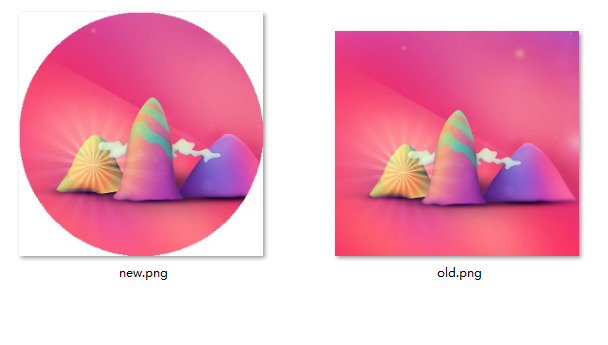## PHP实现将图片或头像转换为圆形（类似微信头像）

•
• 5998
• PHP
• 0
• super_dodo
• 2019/07/03/**
* 将图片转为圆形
* @param [string] \$img 原始图片地址
* @param [string] \$save_img 生成后的圆形图片存储路径
*/
function circular_img(\$img,\$save_img){
\$ext=pathinfo(\$img);
\$src_img = null;
switch (\$ext['extension']) {
case 'jpg':
\$src_img=imagecreatefromjpeg(\$img);
break;
case 'png':
\$src_img=imagecreatefrompng(\$img);
break;
}
\$wh= getimagesize(\$img);
\$w=\$wh;
\$h=\$wh;
\$w=min(\$w,\$h);
\$h= \$w;
\$img = imagecreatetruecolor(\$w, \$h);
//这一句一定要有
imagesavealpha(\$img, true);
//拾取一个完全透明的颜色,最后一个参数127为全透明,并可以设定背景色
\$bg = imagecolorallocatealpha(\$img, 255, 255, 255, 127);
imagefill(\$img, 0, 0, \$bg);
\$r = \$w / 2; //圆半径
\$y_x = \$r; //圆心X坐标
\$y_y = \$r; //圆心Y坐标
for (\$x = 0; \$x < \$w; \$x++) { for (\$y = 0; \$y < \$h; \$y++) { \$rgbColor = imagecolorat(\$src_img, \$x, \$y); if ((((\$x - \$r) * (\$x - \$r) + (\$y - \$r) * (\$y - \$r)) < (\$r * \$r))) { imagesetpixel(\$img, \$x, \$y, \$rgbColor); } } } imagejpeg (\$img,\$save_img,90); imagedestroy(\$img); return true; } \$oldImg = './img/old.png'; //原来的图片 \$newImg = './img/new.png'; //生成的图片 \$res = circular_img(\$oldImg, \$newImg); var_dump(\$res); [/php] #参考网址： #https://www.cnblogs.com/xuweiqiang/p/9956498.html